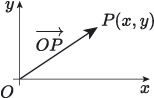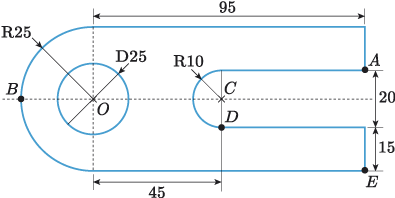Science, Maths & Technology

### Become an OU studentIntroducing vectors for engineering applications

Start this free course now. Just create an account and sign in. Enrol and complete the course for a free statement of participation or digital badge if available.

# 2.4 Position vectors

There is a strong similarity between coordinates and vectors, and we have made use of this similarity when converting between vectors of different forms. We can also make use of this similarity when describing the locations of points in space: the location of a point can be described using a vector.

Let be a point in space. Then the position vector of is a displacement vector where is the origin. This is illustrated in Figure 21.Figure 21 The position vector

The components of the position vector of a point are the same as the coordinates of the point. So the position vector of a point with coordinates is

If a point is denoted by a capital letter, as is the usual convention, then it’s often convenient to denote its position vector by the corresponding lowercase, bold (or underlined) letter. For example, we can denote the position vector of the point by , the position vector of point by , and so on.

## Activity 12

Identify the position vectors of the points labelled to in the following diagram.